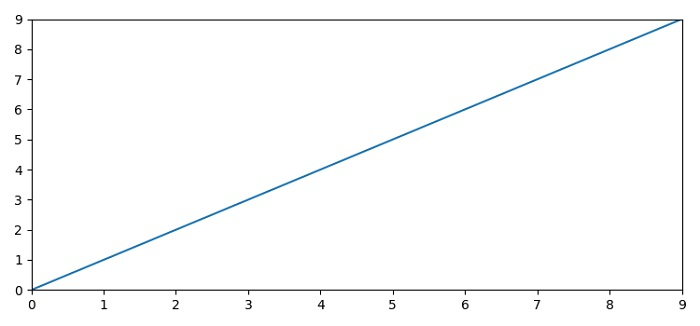# How to make Matplotlib show all X coordinates?

To show all X coordinates (or Y coordinates), we can use xticks() method (or yticks()).

## Steps

• Set the figure size and adjust the padding between and around the subplots.

• Create x and y data points using numpy.

• Set x=0 and y=0 margins on the axes.

• Plot x and y data points using plot() method.

• Use xticks() method to show all the X-coordinates in the plot.

• Use yticks() method to show all the Y-coordinates in the plot.

• To display the figure, use show() method.

## Example

import numpy as np
import matplotlib.pyplot as plt
plt.rcParams["figure.figsize"] = [7.50, 3.50]
plt.rcParams["figure.autolayout"] = True
x = np.arange(0, 10, 1)
y =np.arange(0, 10, 1)
plt.margins(x=0, y=0)
plt.plot(x, y)
plt.xticks(x)
plt.yticks(y)
plt.show()

## Output